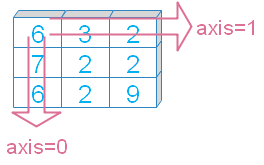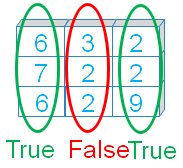# Numpy min()

Numpy

``numpy.min(a,axis=None,out=None,keepdims, initial, where)``
Return min of elements across given axis.
 `a` array, elements to get the min value `axis` Int (optional ), or tuple, default is None, minimum value among all the elements. If axis given then values across the axis is returned. `out` Optional. If given then output to be stored. Must be of same shape as of the output `keepdims` Bool ( Optional ), output matches to the input array dimension. `where` Optional, Elements to include for calculation of minimum value `initial` Optional, int, Minimum value of the output. If given then this is considered if it is less than the actual output
We will use these parameters in our examples.

## Sample array

You can use randint() to create an array for our examples. Or can use fixed elements to create the array.
``````import numpy as np
# my_data=np.random.randint(2,high=7,size=(3,3),dtype='int16')
my_data=np.array([[6, 3, 2], [7, 2, 2], [6, 2, 9]])
print(my_data)``````
Output
``````[[6 3 2]
[7 2 2]
[6 2 9]]``````

## Axis

Minimum value of the elements across the axis.``````print("min()      : ", my_data.min())
print("min(axis=0): ", my_data.min(axis=0))
print("min(axis=1): ", my_data.min(axis=1))``````
Output
``````min()       :  2
min(axis=0) :  [6 2 2]
min(axis=1) :  [2 2 2]``````

## out

Alternative output array, must be of same shape as expected output. Let us first check with axis.
``````x = np.zeros(3,dtype=int)
print(my_data.min(axis=0,out=x))
print(x)``````
Output
``````[6 2 2]
[6 2 2]``````
Without using axis
``````y = np.array(1)
print(my_data.min(out=y))
print(y)``````
Output
``````2
2``````

## keepdims

If it is set to True ( keepdims=True ) then it will take the dimension of input array.
``````print("min(keepdims=True) : ", my_data.min(keepdims=True))
print("min(keepdims=False) : ", my_data.min(keepdims=False))``````
Output
``````min(keepdims=True) :  []
min(keepdims=False) :  2``````

## Using whereBy using where we can say which elements to use and which elements not to use ( by setting True or False ) . While using where we have to give initial value.
``print(my_data.min(where=[True, False,True],initial=2))``
Output
``2``
Using axis with where
``````print(my_data.min(where=[True, False,True],initial=2))
print(my_data.min(axis=1,where=[True, False,True],initial=1))
print(my_data.min(axis=1,where=[True, False,True],initial=10))
print(my_data.min(axis=0,where=[True, False,True],initial=3))``````
Output
``````2
[1 1 1]
[2 2 6]
[3 3 2]``````

## initial

We can assign initial value to our output. Note that the final output will be minimum of initial vlaue and actual min value ( without the initial value ).
``````print(my_data.min())  # 2
print(my_data.min(initial=1)) # 1
print(my_data.min(initial=12)) # 2 ``````
Output
``````2
1
2``````
Check the where option above. The value assigned to intial value is given as output where it is less than the actual output.

## Intial vlaue with Axis option

Compare the values with initial value and without intitial value. We used axis=0 , you can try with axis=1.
``````print(my_data.min(axis=0))  # [6 2 2]
print(my_data.min(axis=0,initial=12)) # [6 2 2]
print(my_data.min(axis=0,initial=1)) # [1 1 1]``````
Output
``````[6 2 2]
[6 2 2]
[1 1 1]``````

Subscribe to our YouTube Channel here

## Subscribe

* indicates required
Subscribe to plus2netplus2net.com